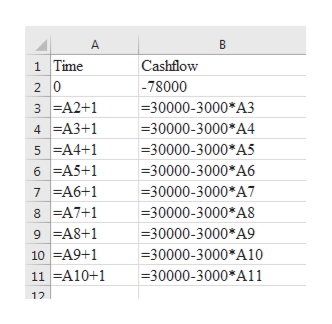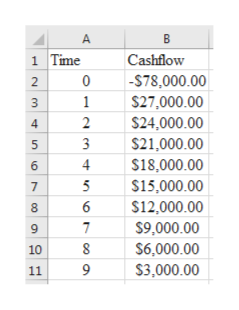# TransITRI is a transportation company with a recent need of a new construction equipment at a first cost of \$78,000 (Equation (1)), zero salvage value, and a cash flow before taxes (CFBT) per year t that follows Equation (2).                                            CFBT = \$30,000 – 3,000t (for t = 1, 2, … T)                                       (1)                                            CFBT = - \$78,000 (for t =0)                                                                  (2)Plot the cash flow diagram from year 0 (t=0) to year 9 (t=9)

Question
8 views
1. TransITRI is a transportation company with a recent need of a new construction equipment at a first cost of \$78,000 (Equation (1)), zero salvage value, and a cash flow before taxes (CFBT) per year t that follows Equation (2).

CFBT = \$30,000 – 3,000t (for t = 1, 2, … T)                                       (1)

CFBT = - \$78,000 (for t =0)                                                                  (2)

Plot the cash flow diagram from year 0 (t=0) to year 9 (t=9)

check_circle

Step 1

The calculation of different cashflows for different years is shown in below table:help_outlineImage Transcriptionclose1 Time Cashflow -78000 3 =A2+1 4 =A3+1 5 =A4+1 =30000-3000*A3 =30000-3000*A4 =30000-3000*A5 6 =A5+1 7 =A6+1 8 =A7+1 9 =A8+1 =30000-3000*A6 =30000-3000*A7 =30000-3000*A8 =30000-3000*A9 10 =A9+1 11 =A10+1 =30000-3000*A10 =30000-3000*A11 12 fullscreen
Step 2

The resultant figures are s...help_outlineImage Transcriptionclose1 Time Cashflow -S78,000.00 \$27,000.00 2 3 \$24,000.00 4 3 \$21,000.00 4 \$18,000.00 5 \$15,000.00 \$12,000.00 \$9,000.00 \$6,000.00 10 \$3,000.00 11 fullscreen

### Want to see the full answer?

See Solution

#### Want to see this answer and more?

Solutions are written by subject experts who are available 24/7. Questions are typically answered within 1 hour.*

See Solution
*Response times may vary by subject and question.
Tagged in